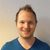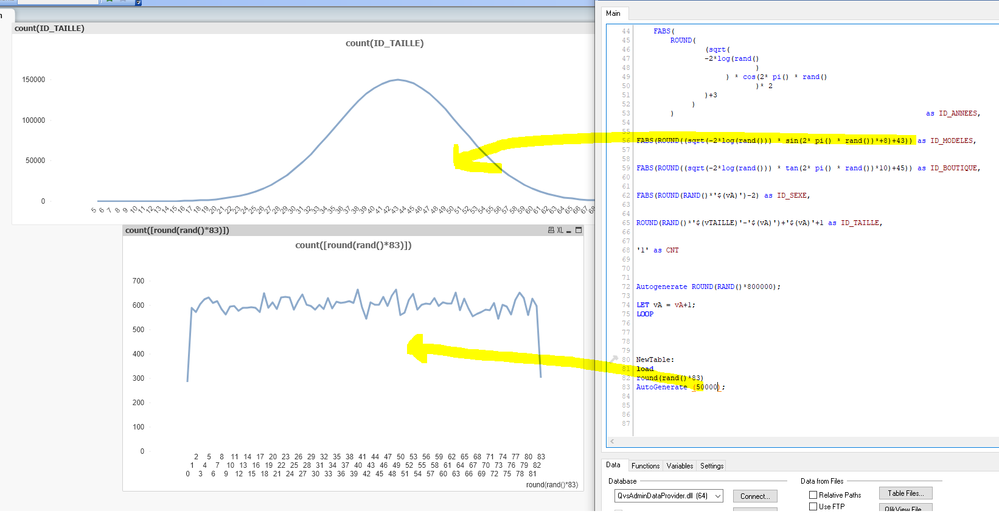# App Development

Announcements
Support Cases coming to Qlik Community Oct. 4! Start chats, open cases, explore resources: READ DETAILS
cancel
Showing results for
Did you mean:Contributor II

## Random function

Hi,

I would like to generate random rows on shoe sales but when I use the random function it returns me a linear distribution. When I load my data, I will always get the same ranking order of shoe brands.

Below is my code:

LET vJOURS = '25' ;
LET vMOIS = '7' ;
LET vANNEES = '5' ;
LET vMODELE = '40' ;
LET vBOUTIQUE = '44' ;
LET vAGE = '45' ;
LET vPAYS = '5' ;
LET vVILLE = '2' ;
LET vCOULEUR = '3' ;
LET vTAILLE = '32' ;
LET vPRIX = '81' ;
LET vA = 0;

DO WHILE vA < 5

Ventes :
ROUND(RAND()*'\$(vJOURS)'+'\$(vA)')+1 as JOURS,
ROUND(RAND()*'\$(vMOIS)')+'\$(vA)'+1 as MOIS,
FABS(ROUND((sqrt(-2*log(rand())) * cos(2* pi() * rand())* 2)+3)) as ID_ANNEES,
FABS(ROUND((sqrt(-2*log(rand())) * sin(2* pi() * rand())*+8)+43)) as ID_MODELES,
FABS(ROUND((sqrt(-2*log(rand())) * tan(2* pi() * rand())*10)+45)) as ID_BOUTIQUE,
FABS(ROUND(RAND()*'\$(vA)')-2) as ID_SEXE,
ROUND(RAND()*'\$(vTAILLE)'-'\$(vA)')+'\$(vA)'+1 as ID_TAILLE,
'1' as CNT
Autogenerate ROUND(RAND()*800000);

LET vA = vA+1;
LOOP

Do you have a solution to this problem so that I can get a real random distribution?

Lucie

Labels (2)

• ### random list values

6 RepliesContributor III

Hi Lucie

What should the results be like? At first glance it looks really, maybe overly, complicated.Contributor II
Author

Hi Marijn,

I would like to get a non-linear distribution of sales when I load data, which means to obtain a classification different from the previous one each time I load the data. But currently, I still find myself with the same ranking of the best sales of shoe brands, only the values(turnover, number of sales, ...) change. I would also like that by filtering on a country, the ranking differs from one country to another. However, in my case, the ranking of the best sales of shoes is always the same regardless of the country, which does not reflect the reality.

ThanksPartner

@_Lp  try code below

SET vA =1;
DO WHILE vA < 5

Ventes :
ROUND(RAND()*'\$(vJOURS)'+'\$(vA)')+1 AS JOURS,
ROUND(RAND()*'\$(vMOIS)')+'\$(vA)'+1 AS MOIS,
FABS(ROUND((SQRT(-2*LOG(RAND())) * COS(2* PI() * RAND())* 2)+3)) AS ID_ANNEES,
FABS(ROUND((SQRT(-2*LOG(RAND())) * SIN(2* PI() * RAND())*+8)+43)) AS ID_MODELES,
FABS(ROUND((SQRT(-2*LOG(RAND())) * TAN(2* PI() * RAND())*10)+45)) AS ID_BOUTIQUE,
FABS(ROUND(RAND()*'\$(vA)')-2) AS ID_SEXE,
ROUND(RAND()*'\$(vTAILLE)'-'\$(vA)')+'\$(vA)'+1 AS ID_TAILLE,
ROWNO() AS CNT
AUTOGENERATE ROUND(RAND()*800000);

LET vA = vA+1;
LOOPContributor II
Author

Hi Gabriel,

I have tried this method before and it doesn't work.

ThanksMVP & Luminary

Here a few ideas how the generic values might be get more/broader distributed:

pow(rand() * sqrt(recno()), rand() * 7)

- MarcusContributor III

I would use a way less complex fomula for randomness: round(rand()*83) for ID_MODELES.

You'd end up with a distribution like this:Tags
Community Browser